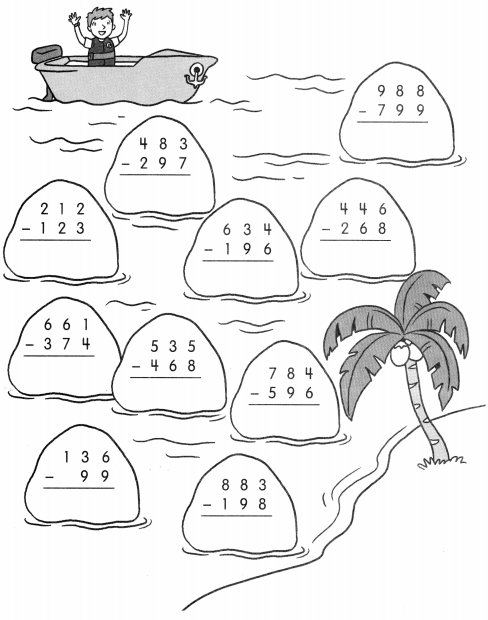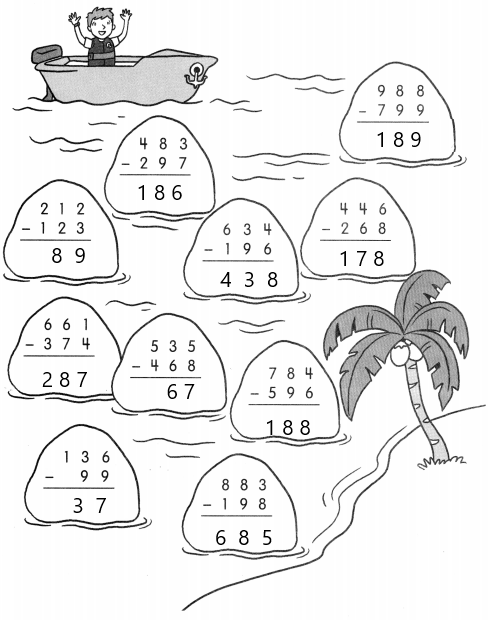# Math in Focus Grade 2 Chapter 3 Practice 7 Answer Key Subtraction with Regrouping in Hundreds, Tens, and Ones

Go through the Math in Focus Grade 2 Workbook Answer Key Chapter 3 Practice 7 Subtraction with Regrouping in Hundreds, Tens, and Ones to finish your assignments.

## Math in Focus Grade 2 Chapter 3 Practice 7 Answer Key Subtraction with Regrouping in Hundreds, Tens, and Ones

Regroup.
Then subtract.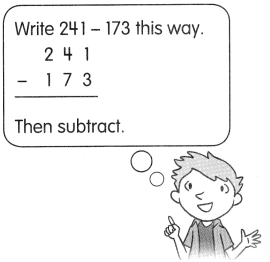Question 1.
241 – 173 = ?
241 – 173
= 2 hundreds 4 tens 1 one – 1 hundred 7 tens 3 ones
= 2 hundreds ________ tens 11 ones – 1 hundred 7 tens 3 ones
= _________ hundred 13 tens 11 ones – 1 hundred 7 tens 3 ones
= _________ hundreds _________ tens _________ ones
241 – 173 = ____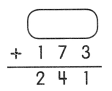241-173 = 68,
173+68 = 241.

Explanation:
Given that 241 – 173 which is 68. So to check the answer we will perform addition, which is 173+68 = 241.
241 – 173
= 2 hundreds 4 tens 1 one – 1 hundred 7 tens 3 ones
= 2 hundreds 3 tens 11 ones – 1 hundred 7 tens 3 ones
= 1 hundred 13 tens 11 ones – 1 hundred 7 tens 3 ones
= 0 hundreds 6 tens 8 ones.

Question 2.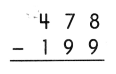478 – 199 = 279,
279+199 = 478.

Explanation:
Given that 478 – 199 which is 279. So to check the answer we will perform addition, which is
279+199 = 478.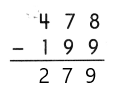Question 3.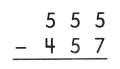555-457 = 98,
457+98 = 555.

Explanation:
Given that 555 – 457 which is 98. So to check the answer we will perform addition, which is
457+98 = 555.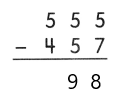Question 4.
924 – 886 = ___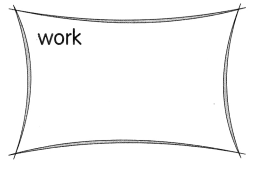924-886 = 38,
38+886 = 924.

Explanation:
Given that 924-886 which is 38. So to check the answer we will perform addition, which is
38+886 = 924.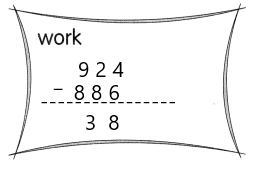Question 5.
818 – 669 = ___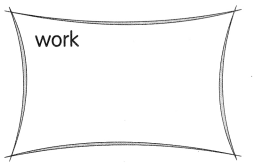818-669 = 149,
149+669 = 818.

Explanation:
Given that 818-669 which is 149. So to check the answer we will perform addition, which is
149+669 = 818.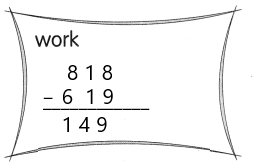Help Daryl ride past these rocks to reach the shore. Subtract and write the correct answer on each rock.

Question 6.